﻿ 题解 UVA11361 【Investigating Div-Sum Property】 – Hzao's Blog

# 题解 UVA11361 【Investigating Div-Sum Property】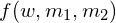表示有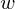位数（可以有前导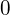）需要确定，需要让各位数字之和除以的余数为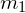，需要让这位数组成的数字除以的余数为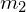需要的方案数。然后我们一步一步确定前面几位数，加起来得到答案。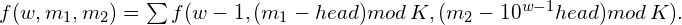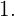记忆化搜索，那么时间复杂度为可是这么大怎么办？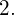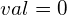时答案为不为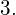可以先让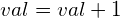，然后紧贴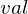求解答案，需要同时记录已经有的各位数字之和和总的数字之和。

#include<cmath>
#include<cstdio>
#include<cstring>
template<typename T>
x=0;char c;T  f=1;
do{c=getchar();if(c=='-')f=-1;else if(c==EOF)return false;}while(c>'9'||c<'0');
do{x=x*10+c-'0';c=getchar();}while(c>='0'&&c<='9');
return x*=f,true;
}
long long A,B,K;
long long tp;//tp[i]表示10 的i次方
long long Div;
long long d;

#define res1 (d[w][m1][m2])
long long f(int w,int m1,int m2){
if(w==0){
return m1==0&&m2==0;
}
if(~d[w][m1][m2])return d[w][m1][m2];
res1=0;
for(register int  i=0;i<=9;++i){
res1+=f(w-1,(m1-i%K+K)%K,(m2-(1ll*tp[w-1]*i%K)+K)%K);
//必须保证mod K之前为非负数 所以加上一个K
}
return res1;
}
long long Getws(long long val){//分割这个数，并且返回它的位数

if(!val){//其实这步可以不要。毕竟在GetAns()的入口处特判了0的情况
return Div=(Div=0)+1;
}
Div=0;
while(val){
Div[++Div]=val%10;
val/=10;
}
return  Div;
}
long long GetAns(long long val){
if(!val)return 1ll;
long long res=0;
++val;
//紧贴val来计算答案，如果不加1会漏掉val这个数
//当然也可以不加1，最后特判val这个数
long long ws=Getws(val);
if(ws*9<K)return 1ll;
long long presum=0,presum2=0;
//presum：当前累计的各位数字之和 如322的presum=3+2+2=7
//presum2：当前已确定数字组成的数：如32***的presum2=32000.
for(register int  i=ws;i>=1;--i){
for(register int  j=0;j<Div[i];++j){
if(j)presum+=1;presum%=K;
if(j)presum2+=tp[i-1];presum2%=K;
//presum,presum2每步取模更方便
res+=f(i-1,(K-presum+K)%K,(K-presum2+K)%K);
//此处有减法，必须保证它是非负数
}
//紧贴val来取值，则最高一位没有被讨论到
//例如，如果val=32565
//如果已经枚举到第二位
//那么实际上我们暂时只会先考虑30***和31***的情况
//但在后面的讨论中，应保证第二位为2
//所以还要把2的贡献累加进去
if(Div[i]){
++presum;
presum2+=tp[i-1];
}
}
return res;
}
void  work(){
long long ans=GetAns(B)-GetAns(A-1);
printf("%lld\n",ans);
}
int main(){
//freopen("testin.txt","r",stdin);
//freopen("testout.txt","w",stdout);
tp=1;
for(register int  i=1;i<=15;++i){
tp[i]=10ll*tp[i-1];
}
long long T;
while(T--){
memset(d,-1,sizeof(d));
work();
}

return 0;
}

## 加入对话

1.2.2条评论

1. 为啥代码块没高亮哇？

1.Hzao说：

因为没找到一个合适的插件…前两天我还发帖子求助来着，有位大佬提供了个方法，但还没来得及试。